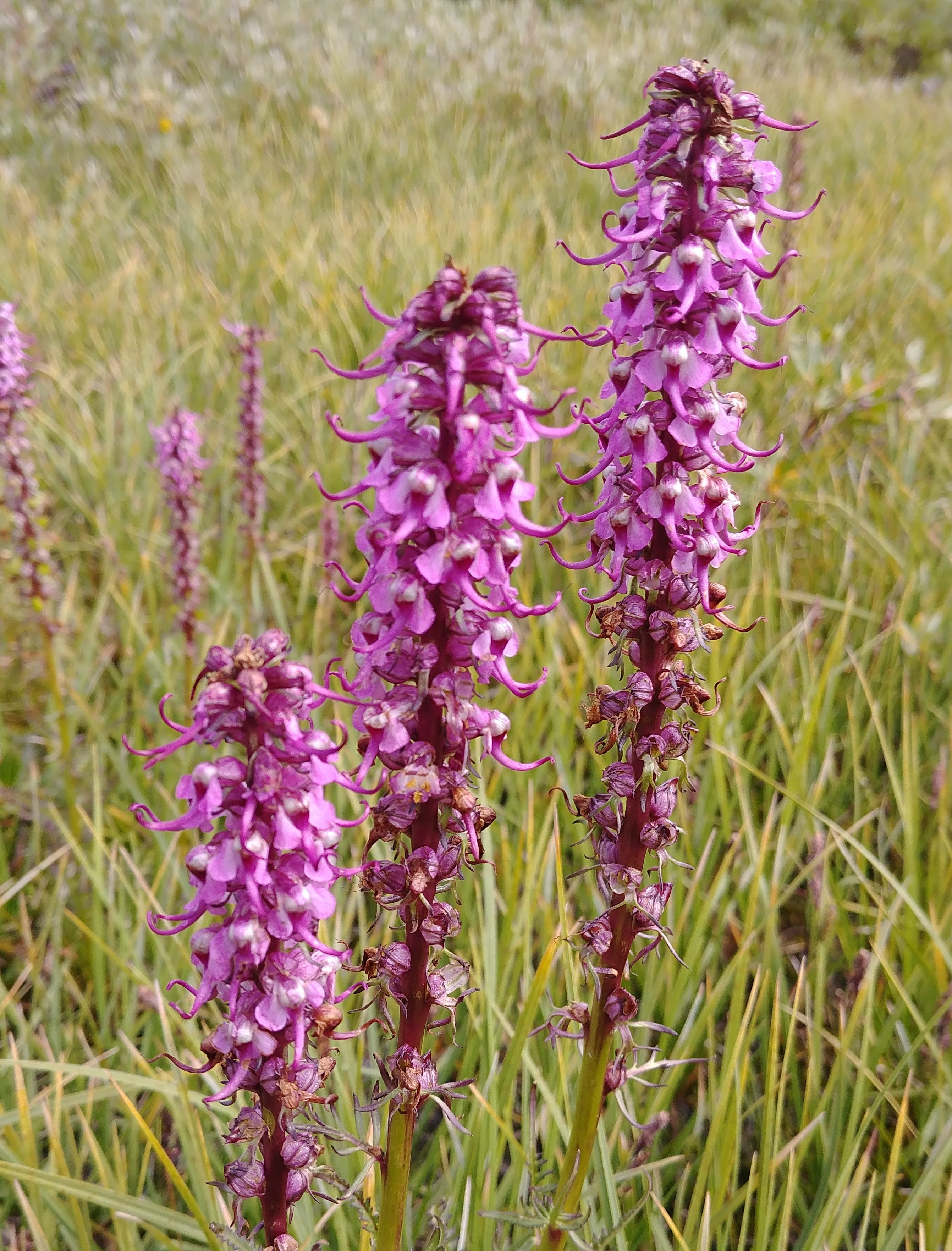# Wetlands and landscapes

Data science, water, isotopes, and ecosystems

## Hello Hugo (and blogdown)

Just a quick post using blogdown and Hugo.This is just a quick post using some blogdown and Hugo functionalities. First I’m going to load some typical information I use for most of my knitted html documents:

``````knitr::opts_chunk\$set(
echo = TRUE,
out.width = "100%"
)
library(tidyverse)``````
``## Warning: package 'tidyverse' was built under R version 3.6.2``
``## Warning: package 'tidyr' was built under R version 3.6.2``
``## Warning: package 'dplyr' was built under R version 3.6.2``

Now, I want to see if I can plot stuff:

``````data(mtcars)
mtcars %>%
mutate(cyl = as.character(cyl)) %>%
ggplot(aes(mpg, hp, color = cyl)) +
geom_point(size = 3) +
theme_bw() +
scale_color_brewer(palette = "Set2")``````Hey, it works! But can I plot something and make it a thumbnail for the post?

``````data(iris)
iris %>%
ggplot(aes(Sepal.Length, Sepal.Width, color = Species)) +
geom_point(size = 3) +
geom_smooth(aes(fill = Species), alpha = 0.8) +
theme_bw() +
scale_color_brewer(palette = "Set1") +
scale_fill_brewer(palette = "Set1")``````
``## `geom_smooth()` using method = 'loess' and formula 'y ~ x'``Well isn’t that neat!